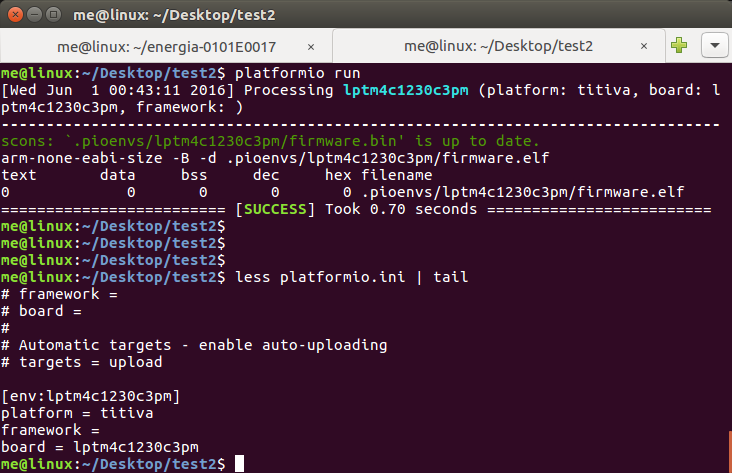# EK-TM4C123GXL: After compilation, firmware.elf has no code

I have written a simple led blinking program for lptm4c1230c3pm controller (EK-TM4C123GXL board). And I didn’t use any framework. After successful (it says) compilation of the program, the firmware.elf file has no text, bss, data, dec or any hex content.
Following is the snapshot of compilation and the platformio.ini content.Please look at the last three lines of compilation result. And below is my led blink program (src/main.c).

``````#define GPIO_PORTF_DIR_R        (*((volatile unsigned int *)0x40025400))
#define GPIO_PORTF_DEN_R        (*((volatile unsigned int *)0x4002551C))
#define GPIO_PORTF_AFSEL_R      (*((volatile unsigned int *)0x40025420))
#define GPIO_PORTF_PUR_R        (*((volatile unsigned int *)0x40025510))
#define GPIO_PORTF_DEN_R        (*((volatile unsigned int *)0x4002551C))
#define GPIO_PORTF_PCTL_R       (*((volatile unsigned int *)0x4002552C))
#define SYSCTL_RCGCGPIO_R       (*((volatile unsigned int *)0x400FE608))
#define SYSCTL_PRGPIO_R         (*((volatile unsigned int *)0x400FEA08))
#define GPIO_PORTF_AMSEL_R      (*((volatile unsigned int *)0x40025528))

#define PF2   (*((volatile unsigned int *)0x40025010))
#define FACTOR 10000

int k, l;

// Make PF2 an output, enable digital I/O, ensure alt. functions off
void SSR_Init(void){
SYSCTL_RCGCGPIO_R |= 0x20;        // 1) activate clock for Port F
while((SYSCTL_PRGPIO_R&0x20)==0){}; // allow time for clock to start
// 2) no need to unlock PF2
GPIO_PORTF_PCTL_R &= ~0x00000F00; // 3) regular GPIO
GPIO_PORTF_AMSEL_R &= ~0x04;      // 4) disable analog function on PF2
GPIO_PORTF_DIR_R |= 0x04;         // 5) set direction to output
GPIO_PORTF_AFSEL_R &= ~0x04;      // 6) regular port function
GPIO_PORTF_DEN_R |= 0x04;         // 7) enable digital port
}

// Make PF2 high
void SSR_On(void){
PF2 = 0x04;
}

// Make PF2 low
void SSR_Off(void){
PF2 = 0x00;
}

void led_delay(int factor)
{
int i, j;
for (i = 0; i < factor; i++)
for (j = 0; j < 1000; j++);
}

int main(void){
SSR_Init();               // initialize PF2 and make it output
while(1){
SSR_On();
led_delay(FACTOR);
SSR_Off();
led_delay(FACTOR);
}
}``````

You need to specify `framework = energia`. We will use only TI `driverlib` from the Energia framework. See:

Thank you @ivankravets . It worked. But I am facing the same clocking (delay won’t work) issue as him.